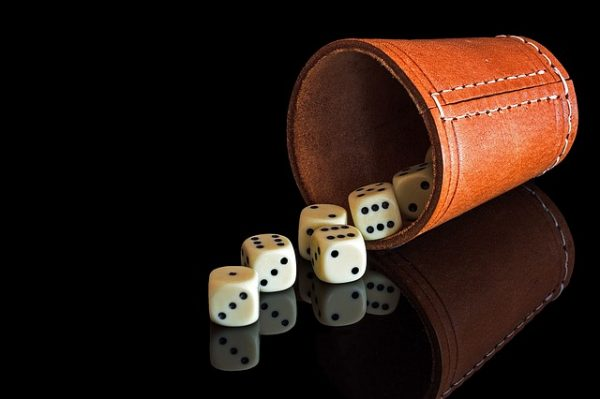## Expert GRE Probability Review: Formulas, Tips, and PracticeWhat’s the probability of getting a perfect GRE Quant score? While you don’t have to be a math whiz to score highly on the GRE, you do need to be able to understand the basic concepts being tested, one of which is probability. Knowing what kind of GRE probability questions to expect will give you a little more confidence on Quant — and help raise your Quant score!

In this extensive GRE probability review, we’ll start by covering what probability is and the ways it’s presented on the GRE. Then, we’ll teach you all of the basic rules and formulas you need to know as well as give you tips for studying probability and approaching GRE probability questions on test day.